# How to drop rows in Pandas DataFrame by index labels?

• Difficulty Level : Expert
• Last Updated : 11 Jun, 2021

Pandas provide data analysts a way to delete and filter data frame using .drop() method. Rows can be removed using index label or column name using this method.

Syntax:
DataFrame.drop(labels=None, axis=0, index=None, columns=None, level=None, inplace=False, errors=’raise’)
Parameters:
labels: String or list of strings referring row or column name.
axis: int or string value, 0 ‘index’ for Rows and 1 ‘columns’ for Columns.
index or columns: Single label or list. index or columns are an alternative to axis and cannot be used together.
level: Used to specify level in case data frame is having multiple level index.
inplace: Makes changes in original Data Frame if True.
errors: Ignores error if any value from the list doesn’t exists and drops rest of the values when errors = ‘ignore’
Return type: Dataframe with dropped valuesNow, Let’s create a sample dataframe

## Python3

 `# import pandas library``import` `pandas as pd`` ` `# dictionary with list object in values``details ``=` `{``    ``'Name'` `: [``'Ankit'``, ``'Aishwarya'``, ``'Shaurya'``,``'Shivangi'``],``    ``'Age'` `: [``23``, ``21``, ``22``,``21``],``    ``'University'` `: [``'BHU'``, ``'JNU'``, ``'DU'``, ``'BHU'``],``}`` ` `# creating a Dataframe object``df ``=` `pd.DataFrame(details,columns ``=` `[``'Name'``,``'Age'``,``'University'``],``                  ``index ``=` `[``'a'``, ``'b'``, ``'c'``, ``'d'``])`` ` `df`

Output: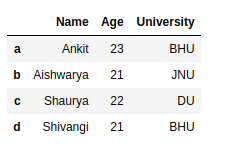Example #1: Delete a single Row in DataFrame by Row Index Label

## Python3

 `# import pandas library``import` `pandas as pd` `# dictionary with list object in values``details ``=` `{``    ``'Name'` `: [``'Ankit'``, ``'Aishwarya'``, ``'Shaurya'``, ``'Shivangi'``],``    ``'Age'` `: [``23``, ``21``, ``22``, ``21``],``    ``'University'` `: [``'BHU'``, ``'JNU'``, ``'DU'``, ``'BHU'``],``}` `# creating a Dataframe object``df ``=` `pd.DataFrame(details, columns ``=` `[``'Name'``, ``'Age'``, ``'University'``],``                  ``index ``=` `[``'a'``, ``'b'``, ``'c'``, ``'d'``])` `# return a new dataframe by dropping a``# row 'c' from dataframe``update_df ``=` `df.drop(``'c'``)` `update_df`

Output :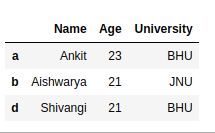Example #2: Delete Multiple Rows in DataFrame by Index Labels

## Python3

 `# import pandas library``import` `pandas as pd` `# dictionary with list object in values``details ``=` `{``    ``'Name'` `: [``'Ankit'``, ``'Aishwarya'``, ``'Shaurya'``, ``'Shivangi'``],``    ``'Age'` `: [``23``, ``21``, ``22``, ``21``],``    ``'University'` `: [``'BHU'``, ``'JNU'``, ``'DU'``, ``'BHU'``],``}` `# creating a Dataframe object``df ``=` `pd.DataFrame(details, columns ``=` `[``'Name'``, ``'Age'``, ``'University'``],``                  ``index ``=` `[``'a'``, ``'b'``, ``'c'``, ``'d'``])` `# return a new dataframe by dropping a row``# 'b' & 'c' from dataframe``update_df ``=` `df.drop([``'b'``, ``'c'``])` `update_df`

Output :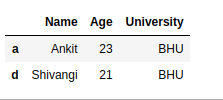Example #3: Delete a Multiple Rows by Index Position in DataFrame

## Python3

 `# import pandas library``import` `pandas as pd` `# dictionary with list object in values``details ``=` `{``    ``'Name'` `: [``'Ankit'``, ``'Aishwarya'``, ``'Shaurya'``, ``'Shivangi'``],``    ``'Age'` `: [``23``, ``21``, ``22``, ``21``],``    ``'University'` `: [``'BHU'``, ``'JNU'``, ``'DU'``, ``'BHU'``],``}` `# creating a Dataframe object``df ``=` `pd.DataFrame(details, columns ``=` `[``'Name'``, ``'Age'``, ``'University'``],``                  ``index ``=` `[``'a'``, ``'b'``, ``'c'``, ``'d'``])` `# return a new dataframe by dropping a row``# 'b' & 'c' from dataframe using their``# respective index position``update_df ``=` `df.drop([df.index[``1``], df.index[``2``]])` `update_df`

Output :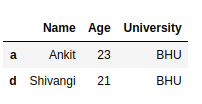Example #4: Delete rows from dataFrame in Place

## Python3

 `# import pandas library``import` `pandas as pd` `# dictionary with list object in values``details ``=` `{``    ``'Name'` `: [``'Ankit'``, ``'Aishwarya'``, ``'Shaurya'``, ``'Shivangi'``],``    ``'Age'` `: [``23``, ``21``, ``22``, ``21``],``    ``'University'` `: [``'BHU'``, ``'JNU'``, ``'DU'``, ``'BHU'``],``}` `# creating a Dataframe object``df ``=` `pd.DataFrame(details, columns ``=` `[``'Name'``, ``'Age'``, ``'University'``],``                  ``index ``=` `[``'a'``, ``'b'``, ``'c'``, ``'d'``])` `# dropping a row 'c' & 'd' from actual dataframe``df.drop([``'c'``, ``'d'``], inplace ``=` `True` `)` `df`

Output :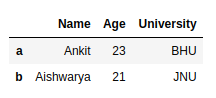My Personal Notes arrow_drop_up